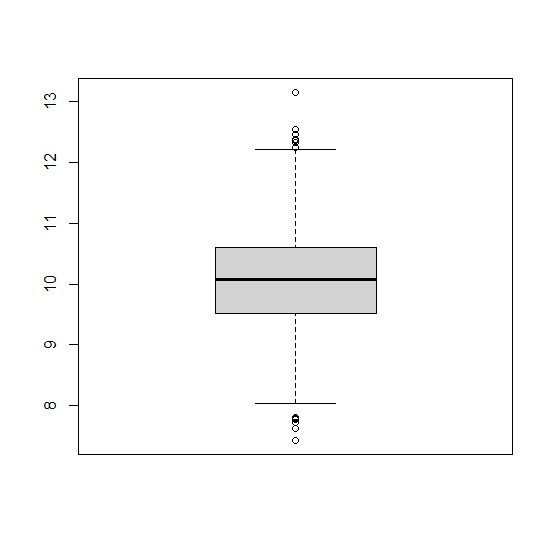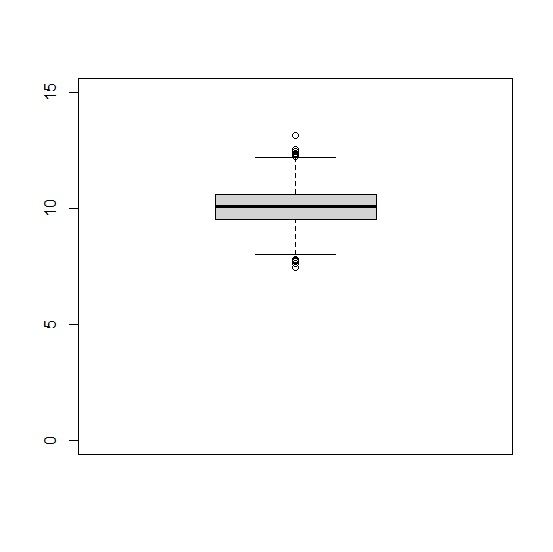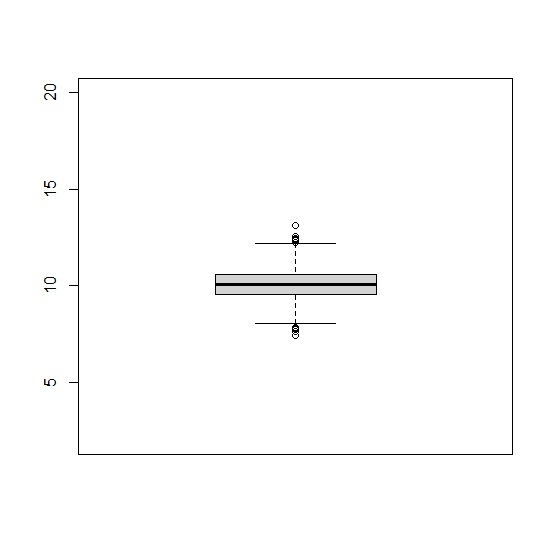# How to set the range for boxplot in base R?

By default, R considers the vector or the data frame column values. But if we want to set the range for boxplot in base R, we can use ylim argument within the boxplot function. For example, if we have a vector called x that contains values starting from 21 to 50 and we want to have the range in the boxplot starting from 1 to 100 then we can use the command

boxplot(x,ylim=c(1,100))

## Example

Live Demo

x<-rnorm(500,10,1)
boxplot(x)

## Output## Example

boxplot(x,ylim=c(0,15))

## Output## Example

boxplot(x,ylim=c(2,20))

## Output